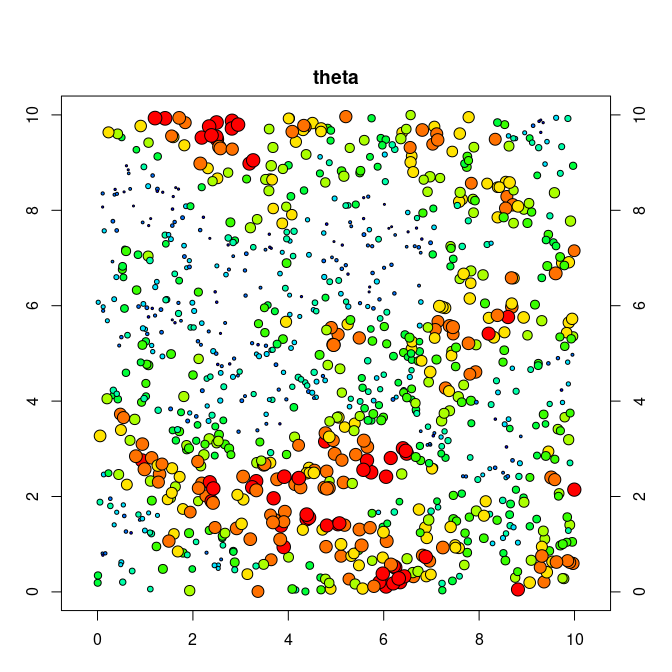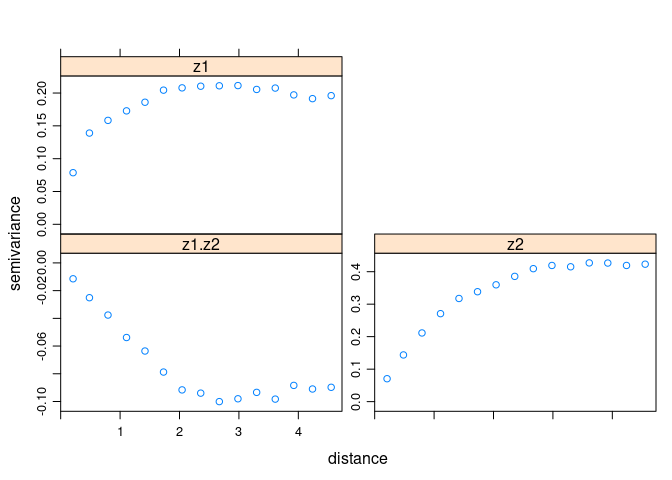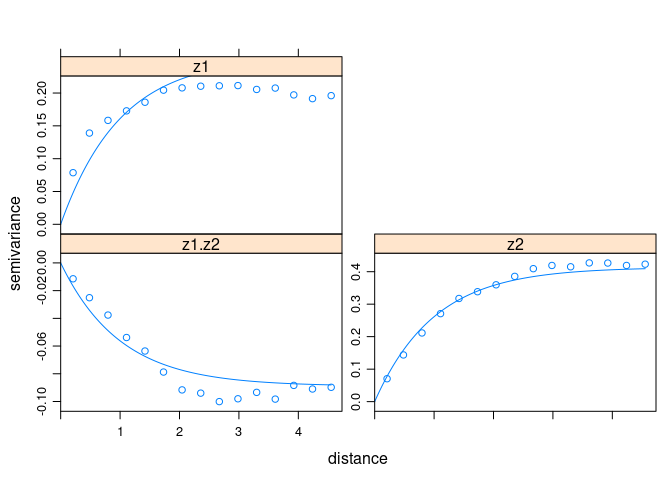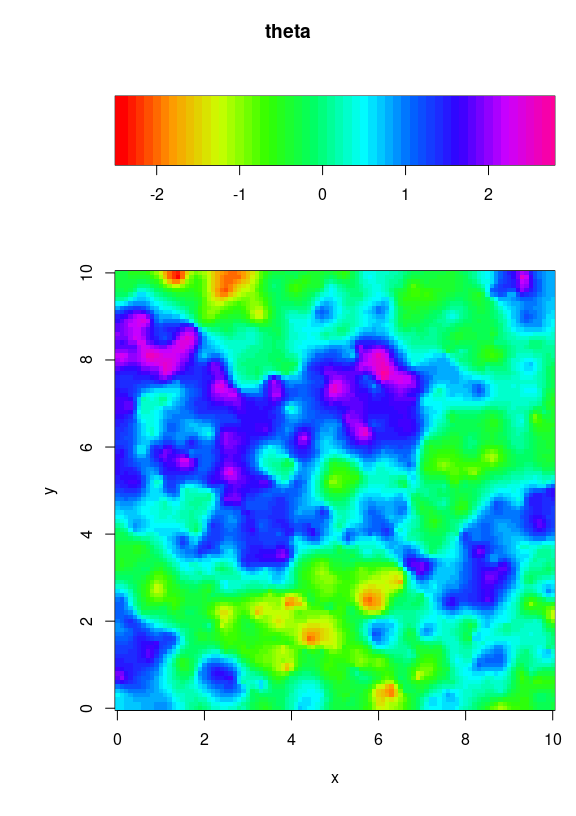# How to register new layer datatypes

## Purpose of this vignette

This vignette explains how to tweak “gmGeostasts” to declare new datatypes for data layers (e.g. for data with special characteristics besides compositional data), and associate creation and prediction function to it. If you are looking for a general introduction to the package, see this other vignette

library(compositions)
#> Welcome to compositions, a package for compositional data analysis.
#> Find an intro with "? compositions"
#>
#> Attaching package: 'compositions'
#> The following object is masked from 'package:gmGeostats':
#>
#>     logratioVariogram
#> The following objects are masked from 'package:stats':
#>
#>     anova, cor, cov, dist, var
#> The following objects are masked from 'package:base':
#>
#>     %*%, norm, scale, scale.default
library(gstat)
#>
#> Attaching package: 'gstat'
#> The following object is masked from 'package:compositions':
#>
#>     fit.lmc
#> The following object is masked from 'package:gmGeostats':
#>
#>     variogram
library(gmGeostats)
library(magrittr)

## Statistical scale and representation functions

The statistical scale of a data layer is a subjective assessment of the way in which pairs of values of that layer need to be compared. Classical statistical scales after Stevens (1946) are the nominal (two values are either equal or they are different), ordinal (two values are either equal, or one is larger than the other), interval (values can be meaningfully compared by the mathematical operation of subtraction) and ratio (values are strictly positive and can be meaningfully compared by the operation of quotient). Other scales have been introduced, such as several compositional scales for data about the amounts and proportions of components forming a system (Aitchison, 1986; van den Boogaart and Tolosana-Delgado, 2013; van den Boogaart, Tolosana-Delgado and Bren, 2021); for circular and spherical data; for distributional data; for positive definite matrices; etc.

A scale $$s$$ is then typically described by a way of computing the difference between two values $$d_s(\cdot, \cdot)$$, coupled with a description of the set $$\mathcal{E}_s$$ of values of that layer that are at all possible. In the case of circular data, the set of possible values is $$[-\pi, \pi)$$, and given the periodicity condition, the way to compare two values $$a$$ and $$b$$ is $$(a-b)$$ modulo $$\pi$$.

To ease the computation with observations $$x$$ on such layers we want to define a transformation $$R(x)$$ that delivers a representation $$z=R(x)$$ of the data, such that: (i) $$R^{-1}(z)$$ exists for all values of the linear span of $$R(x)$$, (ii) it can be ensured that $$R^{-1}(z)\in \mathcal{E}_s$$, and (iii) that $$d_s(x_1, x_2)\approx R(x_1)-R(x_2)$$. A classical representation strategy of circular data is through an embedding into $$R^2$$ the bivariate real space, by means of the transformation $R(\theta)=[\sin(\theta), \cos(\theta)]=\mathbf{z}$ with inverse operation $\theta = \tan^{-1} \frac{z_2}{z_1}$

For our purposes, the absolute minimum you need to program is:

1. choose a class name for your data tyoe (e.g. “periodic”), and create a function with that name taking the values as they might be in your case study, converting them into a datamatrix and giving them the class of your choice
circular = function(x, varname ="theta", conversion=pi/180){
# output to be a (N, 1)-datamatrix
if(length(dim(x))!=2){ # case x is a vector
y = t(t(x))
colnames(y) = varname
}else if(nrow(x)!=1){ # case x is a too large matrix
y = x[, varname]
}
y = y * conversion
class(y) = "circular"
return(y)
}

(The function can do other things, like in this case, allowing a potential conversion from degrees to radians, or managing several input cases).

1. create method of the function compositions::cdt() implementing the representation for data of your type and returning an rmult object
cdt.circular = function(x, ...){
z = cbind(sin(x), cos(x))
colnames(z) = c("z1", "z2")
return(rmult(z, orig=x))
}

It is important that your cdt() method makes use of the two main arguments of the compositions::rmult() function: z (for the transformed scores) and orig (for the untransformed data).

1. create method of the function compositions::cdtInv() implementing the backrepresentation for data of your type (argument z expects the representation, and orig must be exactly what is set in this example; NOTICE the three dots at compositions:::gsi.orig)
cdtInv.circular = function(z, orig=compositions:::gsi.orig(x),...){
#z = unclass(z)
x = atan2(z[,2], z[,1])
return(circular(x, varname=colnames(orig), conversion = 1))
}

## Geostatistical analysis of scaled data, quick and dirty

With these few lines of programming you could already be able to use “gmGeostats” for your data. To show how, we will generate first a univariate, real-valued random field, take it as if it were circular data (in radians), extract some components out of it, and do a geostatistical analysis with the output.

## model setup
set.seed(333275)
xdt = data.frame(x=0, y=0, z=0) # one point is necesary
vg = vgm(model="Exp", psill=1, nugget=0, range=1) # variogram model
gs = gstat(id="z", formula=z~1, locations = ~x+y , data=xdt, nmax=10, model=vg)
## sample point coordinates
x <- runif(2000, min = 0, max = 10) # values between 0 and 10
Xdt = data.frame(x=x[1:1000], y=x[1001:2000])
# simulate random function
Z = predict(gs, newdata=Xdt, nsim=1)
#> drawing 1 GLS realisation of beta...
#> [using conditional Gaussian simulation]
# select columns
Zdt = Z[,3]
# define and plot data
Zdtc = circular(Zdt, varname = "theta", conversion = 1)
pairsmap(Zdtc, loc=Xdt)Now we can proceed with the analysis. First we create the “gmSpatialModel” containing the transformed data

theta.gg =
make.gmMultivariateGaussianSpatialModel(
data=cdt(Zdtc), coords = Xdt, # always set V="clr" in such cases!
formula = ~1 # for ordinary (co)kriging
)

compute and plot the variogram

theta.vg = gmGeostats::variogram(theta.gg)
plot(theta.vg)and model it, in this case with an exponental of effective range approximately 3, a sill of 0.5, and a nugget close to zero. All ways of modelling variograms are allowed, for instance with “gstat” variograms

theta.md = gstat::vgm(model="Exp", range=1, psill=0.5)
theta.gs = fit_lmc(v=theta.vg, g = theta.gg, model = theta.md)
plot(theta.vg, model=theta.gs$model)Finally we extend the original data container with this model theta.gg = make.gmMultivariateGaussianSpatialModel( data=cdt(Zdtc), coords = Xdt, # always set V="clr" in such cases! formula = ~1, # for ordinary (co)kriging model = theta.gs$model
)

The outcome can then be used for validation, prediction or simulation. Here we do cokriging on the same grid we simulated above

x <- seq(from=0, to=10, by=0.1)
xx = expand.grid(x,x)
colnames(xx) = colnames(Xdt)
ng = KrigingNeighbourhood(nmax = 20, omax=7, maxdist=1)
theta.prds = predict(theta.gg, newdata = xx, pars=ng)
#> starting cokriging
#> Intrinsic Correlation found. Good.
#> [using ordinary cokriging]

and the result be reordered and backtransformed

theta.prds.grid = gsi.gstatCokriging2rmult(theta.prds)
theta.prds.back = backtransform(theta.prds.grid, as = cdt(Zdtc))
summary(theta.prds.back)
#>      theta
#>  Min.   :-2.4153
#>  1st Qu.:-0.2403
#>  Median : 0.3168
#>  Mean   : 0.3677
#>  3rd Qu.: 0.9949
#>  Max.   : 2.7100

Note that the function backtransform() is available in package “compositions” from version 1.0.1-9002, and it expects as the second argument as a transformed data set, typically the original one. To plot the result we might have to program a method for image_cokriged that should take care to fictionally reclass the backtransformed data to “spatialGridRmult” and choose a color sequence appropriate for the periodic nature of the data

image_cokriged.circular = function(x, ...){
class(x) = c("spatialGridRmult", "rmult")
image_cokriged(x, breaks=40, col=rainbow(10), ...)
}
image_cokriged(theta.prds.back, ivar="theta")## An excursion on superclasses

“gmGeostats” uses a mixture of S3 and S4 classes to manage the several kinds of objects, S3 classes mostly preferred for simple configuration objects, models and data elements, and S4 classes mostly in use for large compound spatial models and data containers. S4 classes, though being somewhat more complex to handle and slightly slower, have the advantage to allow for multiple dispatch, which this package extensively uses. S4 classes require its fields (called “slots”) to strictly belong to a specific class. To handle this condition, and at the same time allow for multiple methods of specification, estimation, fitting and prediction of spatial models and random functions, “gmGeostats” provides a series of abstract classes allowing to control that certain fields do contain only certain kinds of objects:

gmNeighbourhoodSpecification contains a description of the neighbourhood of a point during interpolation/simulation.

EmpiricalStructuralFunctionSpecification contains a desciption of empirical structural functions, typically empirical variograms in different formats (e.g. “gstatVariogram” from package “gstat”, or “logratioVariogram” from package “compositions”).

ModelStructuralFunctionSpecification, equivalent to the preceding one, this class contains specifications of models for structural functions (e.g. “variogramModel” or “CompLinModCoReg” for packages “gstat” resp. “compositions”).

gmValidationStrategy describes the way a model should be validated.

gmGaussianSimulationAlgorithm specifies the exact gaussian simulation algorithm to be used, and provides its parameters (e.g. number of bands for Turning Bands).

gmTrainingImage for multipoint statistics (MPS) methods, this abstract class gathers all ways to specify a gridded image.

gmUnconditionalSpatialModel convenience class of the union of “gmTrainingImage” and “gmGaussianModel” (a concrete class containing “ModelStructuralFunctionSpecification” with some extra information), it is thought to contain all future specifications of an unconditional random function, beyond the two members currently contained.

gmMPSParameters, analogous to “gmGaussianSimulationAlgorithm” or “gmValidationStrategy”, this abstract class contains all specifications of MPS algorithms available.

gmSpatialMethodParameters is a large container of both two-point and multipoint methods, i.e. descriptors of specific algorithms and their parameters. This union class should only contain other abstract claases!

The package “methods” provides a way of checking the subclasses and superclasses of any specific class, thanks to the function classesToAM():

classesToAM("gmSpatialMethodParameters", includeSubclasses = TRUE)
#>                              gSMP gmNS gMPS gmVS NULL gmKN gDSP LvOO NfCV .NUL
#> gmSpatialMethodParameters       0    0    0    0    0    0    0    0    0    0
#> gmNeighbourhoodSpecification    1    0    0    0    0    0    0    0    0    0
#> gmMPSParameters                 1    0    0    0    0    0    0    0    0    0
#> gmValidationStrategy            1    0    0    0    0    0    0    0    0    0
#> NULL                            0    1    1    1    0    0    0    0    0    0
#> gmKrigingNeighbourhood          0    1    0    0    0    0    0    0    0    0
#> gmDirectSamplingParameters      0    0    1    0    0    0    0    0    0    0
#> LeaveOneOut                     0    0    0    1    0    0    0    0    0    0
#> NfoldCrossValidation            0    0    0    1    0    0    0    0    0    0
#> .NULL                           0    0    0    0    1    0    0    0    0    0

The matrix contain a 1 if the row class is a subclass of the column class, and 0 otherwise.

## Future work

In future extensions of this vignette we will discuss the way to create own structural functions (variograms) and estimation models/methods adapted to the nature of the data, and register them to the package (usage of setIs() and coercion in conjunction with the abstract classes mention, validate()- and predict()-methods, creation of own make.gm****Model() data containers, etc). We will continue with our illustrative example of circular data, using developments by Wackernagel (2003) and de Iaco et al (2013).